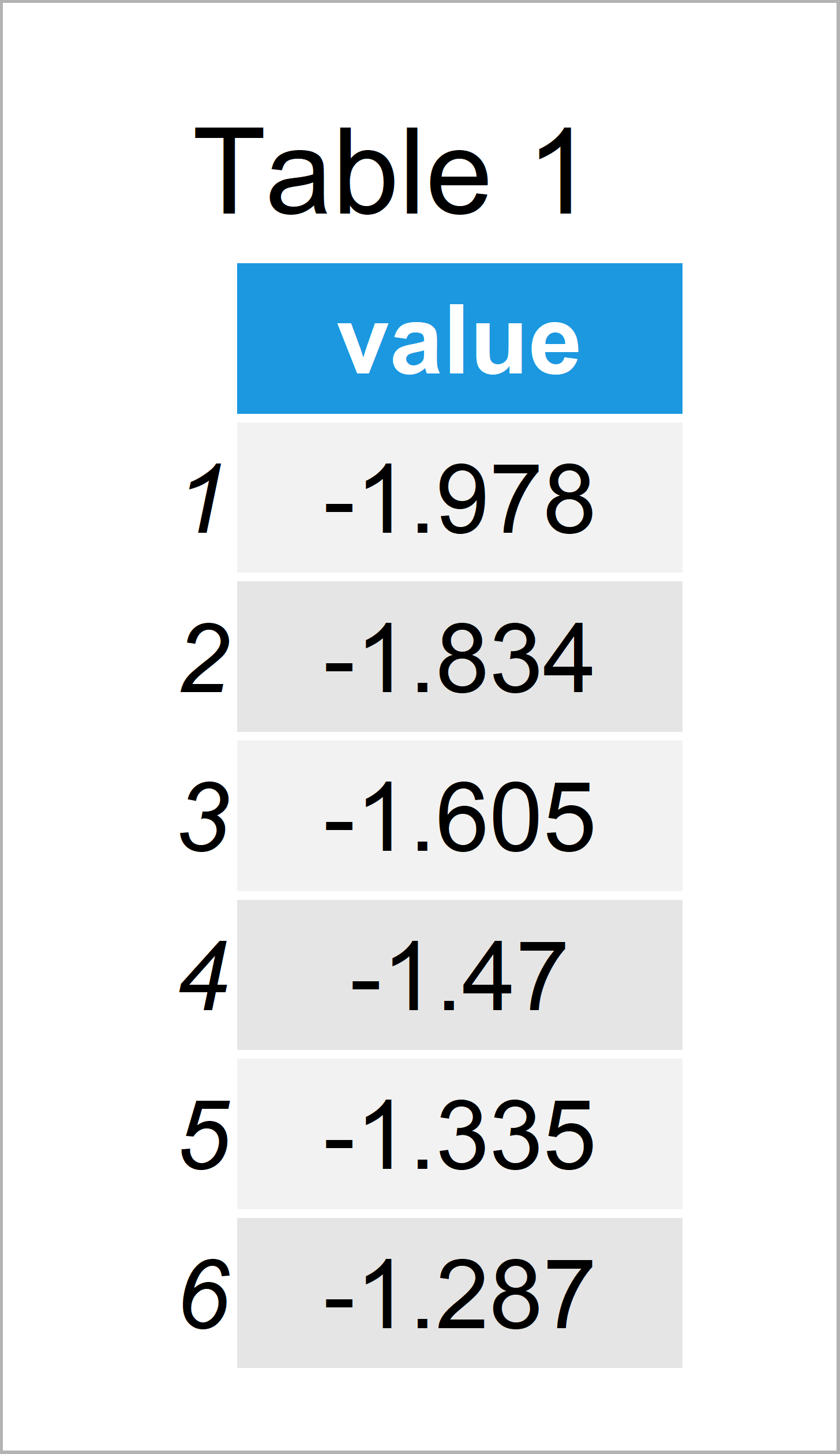# Plot Only One Variable in ggplot2 Plot in R (2 Examples)

This tutorial explains how to draw a ggplot2 scatterplot with only one variable in the R programming language.

## Example Data, Packages & Basic Graph

The first step is to create some data that we can use in the examples below:

```set.seed(563478)                                  # Create example data
data <- data.frame(value = sort(rnorm(100)))Table 1 shows the structure of our example data – It contains 100 observations and only one single column.

In order to use the functions of the ggplot2 package, we also have to install and load ggplot2:

```install.packages("ggplot2")                       # Install ggplot2 package

Next, we may try to use the typical ggplot2 syntax to draw our data:

```ggplot(data, aes(value)) +                        # Try to draw ggplot2 plot
geom_point()
# Error: geom_point requires the following missing aesthetics: y
# Run `rlang::last_error()` to see where the error occurred.```

Unfortunately, the RStudio console returns the error message “Error: geom_point requires the following missing aesthetics: y“.

This error message appears, because we have not specified a second variable to show in our geom_point scatterplot.

So how can we draw a ggplot2 plot with only one variable? You guessed it, that’s what I’ll explain next!

## Example 1: Draw ggplot2 Plot Based On Only One Variable Using ggplot & nrow Functions

In this example, I’ll explain how to use the ggplot and nrow functions to draw a ggplot2 scatterplot with only one variable.

More precisely, we create a sequence from 1 to the number of rows in our data frame and use this sequence as an x-variable.

Consider the following R code:

```ggplot(data, aes(x = 1:nrow(data), y = value)) +  # Apply nrow function
geom_point()```As shown in Figure 1, we have plotted an xy-plot with only one column in our data object.

## Example 2: Draw ggplot2 Plot Based On Only One Variable Using qplot & seq_along Functions

As a second alternative to the previous example, I’ll illustrate how to use the qplot and seq_along functions to draw a ggplot2 scatterplot using only one variable.

Have a look at the following R code:

`qplot(seq_along(data\$value), data\$value)          # Apply qplot function`Note that we had to use the \$ operator to access the value column of our data frame.

This has the disadvantage that we have to call our data frame every time. However, at the same time it has the advantage that we could even draw a vector object that is not stored in a data frame.

## Video, Further Resources & Summary

If you need more information on the R programming code of this tutorial, I recommend having a look at the following video of my YouTube channel. I’m explaining the content of this tutorial in the video:

Please accept YouTube cookies to play this video. By accepting you will be accessing content from YouTube, a service provided by an external third party.If you accept this notice, your choice will be saved and the page will refresh.

In addition, you may want to read the related posts of my website:

This post has illustrated how to draw a single variable in a ggplot2 dotplot in R programming. Don’t hesitate to let me know in the comments below, in case you have further questions.

Subscribe to the Statistics Globe Newsletter